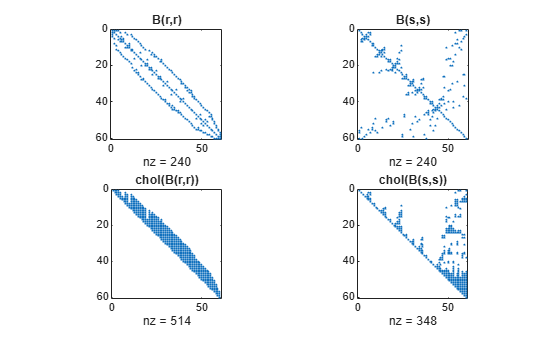# symamd

Symmetric approximate minimum degree permutation

## Syntax

```p = symamd(S) p = symamd(S,knobs) [p,stats] = symamd(...) ```

## Description

`p = symamd(S)` for a symmetric positive definite matrix `S`, returns the permutation vector `p` such that `S(p,p)` tends to have a sparser Cholesky factor than `S`. To find the ordering for `S`, `symamd` constructs a matrix `M` such that ```spones(M'*M) = spones (S)```, and then computes `p = colamd(M)`. The `symamd` function may also work well for symmetric indefinite matrices.

`S` must be square; only the strictly lower triangular part is referenced.

`p = symamd(S,knobs)` where `knobs` is a scalar. If `S` is `n`-by-`n`, rows and columns with more than `knobs*n` entries are removed prior to ordering, and ordered last in the output permutation `p`. If the `knobs` parameter is not present, then `knobs = spparms('wh_frac')`.

`[p,stats] = symamd(...)` produces the optional vector `stats` that provides data about the ordering and the validity of the matrix `S`.

 `stats(1)` Number of dense or empty rows ignored by `symamd` `stats(2)` Number of dense or empty columns ignored by `symamd` `stats(3)` Number of garbage collections performed on the internal data structure used by `symamd` (roughly of size `8.4*nnz(tril(S,-1)) + 9n` integers) `stats(4)` `0` if the matrix is valid, or `1` if invalid `stats(5)` Rightmost column index that is unsorted or contains duplicate entries, or `0` if no such column exists `stats(6)` Last seen duplicate or out-of-order row index in the column index given by `stats(5)`, or `0` if no such row index exists `stats(7)` Number of duplicate and out-of-order row indices

Although, MATLAB® built-in functions generate valid sparse matrices, a user may construct an invalid sparse matrix using the MATLAB C or Fortran APIs and pass it to `symamd`. For this reason, `symamd` verifies that `S` is valid:

• If a row index appears two or more times in the same column, `symamd` ignores the duplicate entries, continues processing, and provides information about the duplicate entries in `stats(4:7)`.

• If row indices in a column are out of order, `symamd` sorts each column of its internal copy of the matrix `S` (but does not repair the input matrix `S`), continues processing, and provides information about the out-of-order entries in `stats(4:7)`.

• If `S` is invalid in any other way, `symamd` cannot continue. It prints an error message, and returns no output arguments (`p` or `stats`).

The ordering is followed by a symmetric elimination tree post-ordering.

## Examples

collapse all

Here is a comparison of reverse Cuthill-McKee and minimum degree on the Bucky ball example mentioned in the `symrcm` reference page.

```B = bucky+4*speye(60); r = symrcm(B); p = symamd(B); R = B(r,r); S = B(p,p); subplot(2,2,1), spy(R,4), title('B(r,r)') subplot(2,2,2), spy(S,4), title('B(s,s)') subplot(2,2,3), spy(chol(R),4), title('chol(B(r,r))') subplot(2,2,4), spy(chol(S),4), title('chol(B(s,s))')```Even though this is a very small problem, the behavior of both orderings is typical. RCM produces a matrix with a narrow bandwidth which fills in almost completely during the Cholesky factorization. Minimum degree produces a structure with large blocks of contiguous zeros which do not fill in during the factorization. Consequently, the minimum degree ordering requires less time and storage for the factorization.

 Davis, Timothy A., John R. Gilbert, Stefan I. Larimore, and Esmond G. Ng. “Algorithm 836: COLAMD, a Column Approximate Minimum Degree Ordering Algorithm.” ACM Transactions on Mathematical Software 30, no. 3 (September 2004): 377–380. https://doi.org/10.1145/1024074.1024080.